Next: General contrast functions Up: One-unit contrast functions Previous: Negentropy

### Higher-order cumulants

Mathematically the simplest one-unit contrast functions are provided by higher-order cumulants like kurtosis (see Appendix A for definition). Denote by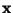the observed data vector, assumed to follow the ICA data model (11). Now, let us search for a linear combination of the observations xi, say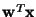, such that its kurtosis is maximized or minimized. Obviously, this optimization problem is meaningful only if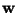is somehow bounded; let us assume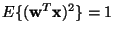. Using the (unknown) mixing matrix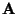, let us define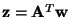. Then, using the data model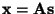one obtains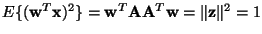(recall that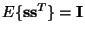), and the well-known properties of kurtosis (see Appendix A) give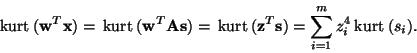(27)

Under the constraint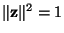, the function (27) has a number of local minima and maxima. To make the argument clearer, let us assume for the moment that in the mixture (11) there is at least one independent component sj whose kurtosis is negative, and at least one whose kurtosis is positive. Then, as was shown in , the extremal points of (27) are the canonical base vectors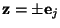, i.e., vectors whose all components are zero except one component which is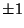. The corresponding weight vectors are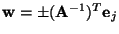, i.e., the rows of the inverse of the mixing matrix, up to a multiplicative sign. So, by minimizing or maximizing the kurtosis in Eq. (27) under the given constraint, one obtains one of the independent components as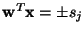. These two optimization modes can also be combined into a single one, because the independent components correspond always to maxima of the modulus of the kurtosis.

Kurtosis has been widely used for one-unit ICA (see, for example, [40,103,71,72]), as well as for projection pursuit . The mathematical simplicity of the cumulants, and especially the possibility of proving global convergence results, as in [40,71,72], has contributed largely to the popularity of cumulant-based (one-unit) contrast functions in ICA, projection pursuit and related fields. However, it has been shown, for example in , that kurtosis often provides a rather poor objective function for the estimation of ICA, if the statistical properties of the resulting estimators are considered. Note that despite the fact that there is no noise in the ICA model (11), neither the independent components nor the mixing matrix can be computed accurately because the independent components si are random variables, and, in practice, one only has a finite sample of. Therefore, the statistical properties of the estimators ofand the realizations of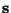can be analyzed just as the properties of any estimator. Such an analysis was conducted in  (see also ), and the results show that in terms of robustness and asymptotic variance, the cumulant-based estimators tend to be far from optimal5. Intuitively, there are two main reasons for this. Firstly, higher-order cumulants measure mainly the tails of a distribution, and are largely unaffected by structure in the middle of the distribution . Secondly, estimators of higher-order cumulants are highly sensitive to outliers . Their value may depend on only a few observations in the tails of the distribution, which may be outliers.Next: General contrast functions Up: One-unit contrast functions Previous: Negentropy
Aapo Hyvarinen
1999-04-23